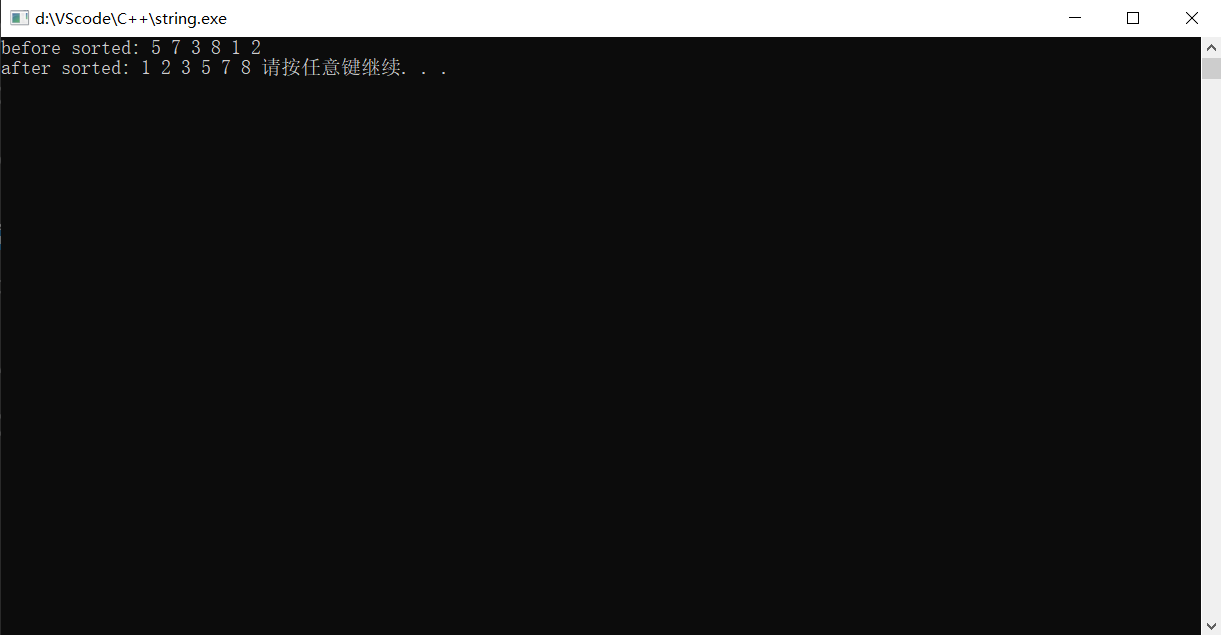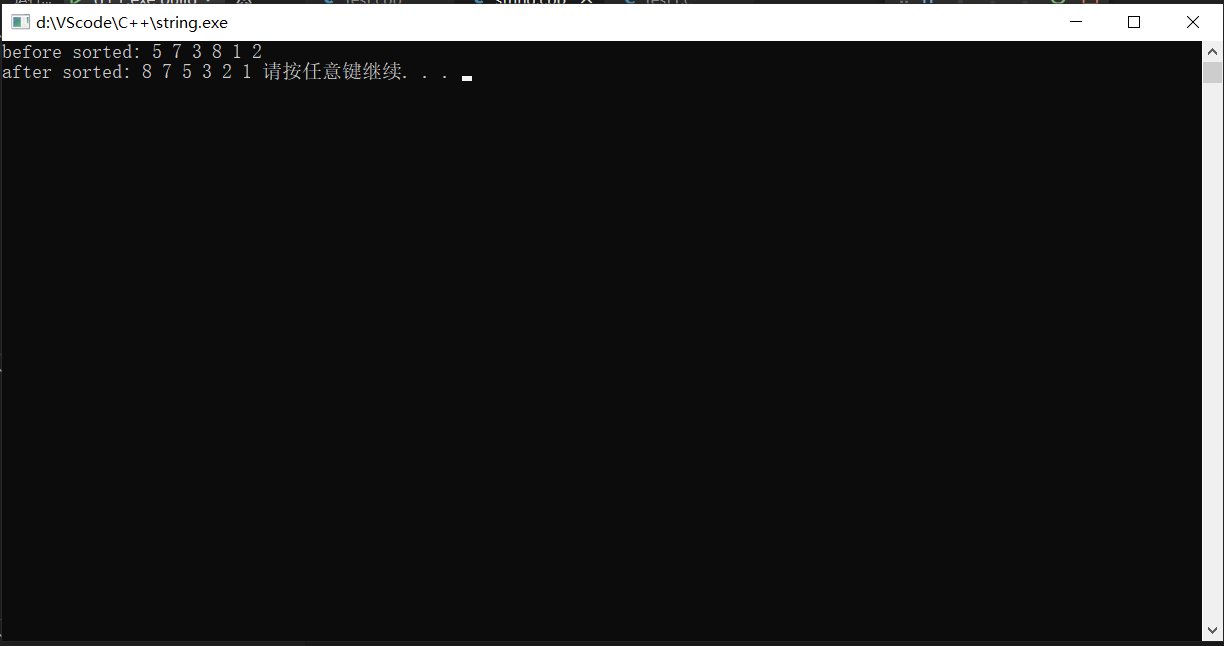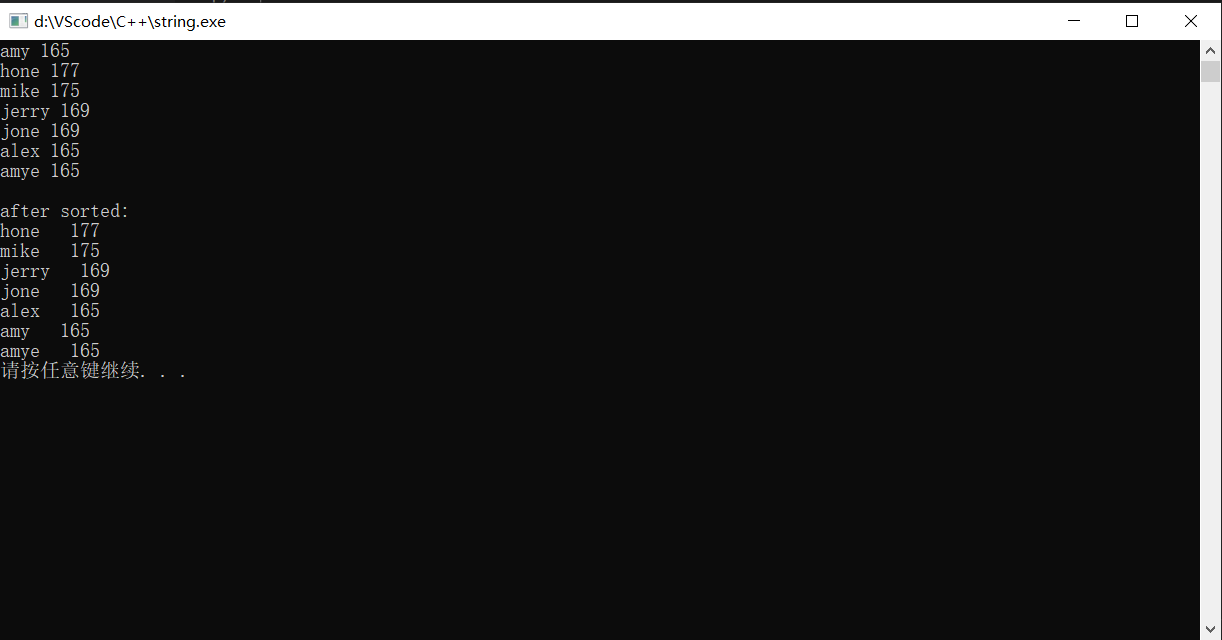• 【C++】vector数组排序

千次阅读 2021-05-23 15:06:02
最近刷力扣的时候遇到了好多vector数组自定义排序问题，这里先来总结一下。 vector是C++的STL中一个重要的容器嘛，STL不用说也知道它的功能十分强大，所以有一些内置排序函数。下面来细说一下。 一.简单升序排序 ...

最近刷力扣的时候遇到了好多vector数组自定义排序问题，这里先来粗略的总结一下。

vector是C++的STL中一个重要的容器嘛，STL不用说也知道它的功能十分强大，所以有一些内置排序函数。下面来细说一下。

一.简单升序排序

（1）准备

vector头文件，用来引入vector数组

algorithm头文件，用来引入sort函数

（2）函数格式

sort(name.begin(),name.end());

name.begin()表示指向数组头的迭代器，name.end()表示指向数组尾下一个位置的迭代器，该式表示将叫name的vector元素按从小到大进行升序排序。

（3）完整代码

#include<iostream>
#include<cstdlib>
#include<algorithm>
#include<vector>
using namespace std;
int main(){
int ans={5,7,3,8,1,2};
vector<int>num;
cout<<"before sorted: ";
for(int i=0;i<6;i++)
{
cout<<ans[i]<<" ";
num.emplace_back(ans[i]);
}
cout<<endl;
cout<<"after sorted: ";
//按升序排序
sort(num.begin(),num.end());
for(int i=0;i<num.size();i++)
{
cout<<num[i]<<" ";
}
system("pause");
return 0;
}

（4）结果二.简单降序排序

（1）函数格式

sort(name.rbegin(),name.rend());

name.rbegin()表示指向数组尾的迭代器，name.rend()表示指向数组头前一个位置的迭代器，该式表示将叫name的vector元素按从大到小进行降序排序。

（2）完整代码

#include<iostream>
#include<cstdlib>
#include<algorithm>
#include<vector>
using namespace std;
int main(){
int ans={5,7,3,8,1,2};
vector<int>num;
cout<<"before sorted: ";
for(int i=0;i<6;i++)
{
cout<<ans[i]<<" ";
num.emplace_back(ans[i]);
}
cout<<endl;
cout<<"after sorted: ";
//按降序排序
sort(num.rbegin(),num.rend());
for(int i=0;i<num.size();i++)
{
cout<<num[i]<<" ";
}
system("pause");
return 0;
}

（3）结果三.自定义函数排序

我们在很多时候都要按照题意来进行排序，而且一般这个对象不止含有一个属性，所以我们需要来自定义它的排序方式。下面以一群学生为例，我们对他们身高进行降序排序，当身高相同时按名字中的字母顺序排序。

学生列表

nameheight
amy165
hone177
mike175
jerry169
jone169
alex165
amye165

（1）函数格式

sort(res.begin(),res.end(),[&](const typename&a,const typename&b)->bool{
return (输入你的排序标准);
});

（2）代码

#include<iostream>
#include<cstdlib>
#include<algorithm>
#include<vector>
#include<string>
#include<unordered_map>
using namespace std;
int main(){
//我们将学生信息存入map中
unordered_map<string,int>map;
string name;
int height;
for(int i=0;i<7;i++)
{
cin>>name>>height;
map[name]=height;
}

//将学生名字存入vector数组中
vector<string>res;
for(auto &[key,value]:map)
{
res.emplace_back(key);
}

//自定义排序，按身高降序，身高相同时则按名字升序排列
sort(res.begin(),res.end(),[&](const string&a,const string&b)->bool{
return map[a]==map[b]?a<b:map[a]>map[b];
});

//输出排列后的顺序
cout<<endl;
cout<<"after sorted: "<<endl;
for(int i=0;i<res.size();i++)
{
cout<<res[i]<<"   "<<map[res[i]]<<endl;
}
system("pause");
return 0;
}

（3）结果展开全文stl 排序 vector
• 对于这样的二维数组我们想要按其某一列的元素的大小进行排列应该怎么解决呢？

我们都用过sort()函数，对于一个vector vec;我们可以很轻松的写出：

//sort()函数默认使用升序排列
sort(vec.begin(),vec.end());

但是当我们碰到vector<vector>时，对于这样的二维数组我们想要按其某一列的元素的大小进行排列应该怎么解决呢？
这个时候就可以重写sort()函数的第三个参数了，具体实现如下：

#include<iostream>
#include<vector>
#include<algorithm>

using namespace std;

class comp
{public:
bool operator()(vector<int> a, vector<int> b)
{
return a < b;
}
};

int main()
{
vector<vector<int>> vec{ {100,200},{200,1300},{1000,1250},{2000,3200} };

sort(vec.begin(), vec.end(), comp());
for (const auto& p : vec)
{
cout << p << "," << p << endl;
}
}

还有一种在sort内定义的方法：

#include<iostream>
#include<vector>
#include<algorithm>

using namespace std;

int main()
{
vector<vector<int>> vec{ {100,200},{200,1300},{1000,1250},{2000,3200} };

sort(vec.begin(), vec.end(), [](const auto& a, const auto& b)
{
return a < b;
});

for (const auto& p : vec)
{
cout << p << "," << p << endl;
}
}

展开全文c++ 开发语言 后端 容器 算法
• sort() #include <iostream>...//时间复杂度n*log2(n)的排序算法，默认升序 void main() { vector<int> p; int a; while (cin >> a) { p.push_back(a); if ('\n' == cin.g.
• sort()
#include <iostream>//输入输出
#include <vector>
#include<algorithm>//sort方法

using namespace std;

//时间复杂度n*log2(n)的排序算法，默认升序
void main() {
vector<int> p;
int a;
while (cin >> a) {
p.push_back(a);
if ('\n' == cin.get()) break;
}
sort(p.begin(), p.end());

for (int i = 0; i < p.size(); i++) cout << p[i] << ' ';
cout << endl;
}

举个栗子：
input：1 7 3 8 5 4
output：1 3 4 5 7 8

此外，sort()是有第三个参数的，自己编写一个比较函数来实现

#include <iostream>//输入输出
#include <vector>
#include<algorithm>

using namespace std;

bool compare_decrease(int a, int b)
{
return a > b;   //从大到小的排列
}

void main() {
vector<int> p;
int a;
while (cin >> a) {
p.push_back(a);
if ('\n' == cin.get()) break;
}
sort(p.begin(), p.end(),compare_decrease);

for (int i = 0; i < p.size(); i++) cout << p[i] << ' ';
cout << endl;
}

举个栗子：
input：1 7 3 8 5 4
output：8 7 5 4 3 1

展开全文c++ 算法
• 一维数组实现 返回值等于0:o1=o2 返回值>0则o1>o2 返回值<0则o1<o2 比较时： o1在前是升序，o2在前是降序 Vector<Integer> comPare = new Vector<>(); comPare.add(3);

1.Comparator接口

源码：

public interface Comparator<T> {
int compare(T o1, T o2);
}

一维数组实现

• 返回值等于0:o1=o2
• 返回值>0则o1>o2
• 返回值<0则o1<o2

比较时：

• o1在前是升序，o2在前是降序
Vector<Integer> comPare = new Vector<>();
comPare.sort(new Comparator<Integer>() {
@Override
public int compare(Integer o1, Integer o2) {
o1在前是升序，o2在前是降序
return o1-o2;
}
});

二维数组的实现

对vector的二维数组进行排序，

• 第2个元素相同，则按第1个元素排升序；
• 否则只按第2个元素排降序。
Vector<Vector<Integer>> vector = new Vector<>();
vector.sort(new Comparator<Vector<Integer>>() {
@Override
public int compare(Vector<Integer> o1, Vector<Integer> o2) {
if (o2.get(1)==o1.get(1))
return o1.get(0)-o2.get(0);
return o2.get(1)-o1.get(1);
}
});

展开全文数据结构
• 排序 头文件：#include <algorithm> 示例如下，默认升序 #include <iostream> #include <vector> #include <algorithm> using namespace std; int main(){ vector<int>vec{5,4,...c++ 数据结构 c语言 stl
• 直接插入法排序vector数组实现（以及"vector subscript out of range"越界问题处理）直接插入法基本原理代码段 直接插入法基本原理 在从小到大的排序过程中，设置一个哨兵位，如果a[ i ] > a[ i - 1 ]（i 从 1 ...排序
• 今天在做下面这道题的时候糊涂了，把二维数组排序做错了。 题目 假设有打乱顺序的一群人站成一个队列，数组 people 表示队列中一些人的属性（不一定按顺序）。每个 people[i] = [hi, ki] 表示第 i 个人的身高为 ...贪心算法 C++ vector 排序 lamda表达式
• java数组排序和索引发布时间：2020-05-27 21:14:36来源：亿速云阅读：151作者：鸽子给定一个排序数组和一个目标值，在数组中找到目标值，并返回其索引。如果目标值不存在于数组中，返回它将会被按顺序插入的位置。你...
• 刷题时，对于多个vector数组按照第二个元素的大小进行排序，其实很简单。 力扣1710. Maximum Units on a Truck 但是一开始代码写的是 class Solution { public: static bool cmp(vector &a,vector&b) { ...c++ 数据结构 排序算法
• 数组排序练习

2021-11-21 22:47:22
定义一个和nums容量相同的vector类并将全部元素初始化为0，遍历nums中的元素，将非零的元素按顺序输入新的容器，即可得到目标数组。 代码如下： class Solution { public: void moveZeroes(vector<int>&...c++ leetcode 排序算法
• 在一个 n * m的二维数组中，每一行都按照从左到右递增的顺序排序，每一列都按照从上到下递增的顺序排序。请完成一个高效的函数，输入这样的一个二维数组和一个整数，判断数组中是否含有该整数。 一、解题思路 从...
• 5938. 找出数组排序后的目标下标 给你一个下标从 0 开始的整数数组 nums 以及一个目标元素 target 。 目标下标 是一个满足 nums[i] == target 的下标 i 。 将 nums 按 非递减 顺序排序后，返回由 nums 中目标下标...c++ leetcode 数据结构
• 问题陈述给定一个由N个不同元素组成的数组，请找到对数组进行排序所需的最小交换次数示例如果array为{4，2，1，3}，则需要2次交换将arr 与arr 交换用arr 交换arr 算法1.在C++中创建一对向量，其中第一个...
• 1. array 定义的时候必须定义数组的元素个数;而vector 不需要；　且只能包含整型字面值常量，枚举常量或者用常量表达式初始化的整型const对象，非const变量以及需要到运行阶段才知道其值的const变量都不能用来定义...
• 对于几种排序算法，尤其是快排，堆排序和归并排序这种，真的是写了忘，忘了写,最近笔试遇到了链表排序，需要用到归并排序，又想起了数组的归并，发现自己还有地方都没有掌握透彻，也遇到了很多坑。 首先需要缕清...数据结构 排序 c++
• vector本来就是可以用来代替一维数组的，vector提供了operator[]函数，可以像数组一样的操作，而且还有边界检查，动态改变大小。这里只介绍用它来代替二维的数组，二维以上的可以依此类推。1、定义二维vectorvector ...
• 文章目录 一维vector排序 二维vector排序 Lambda的语法 Capture（用以访问外部作用域） 问题:今天刷题的时候，需要对二维vector n×2_{n\times 2}n×2​按照第二关键字排序。看到别人使用lambda表达式进行排序，这里...
• vector<int> v; int main() { int x; while(cin>>x) { v.push_back(x); } sort(v.begin(),v.end()); for(vector<int>::iterator it=v.begin(); it!=v.end(); it++) { cout<&l数据结构 STL vector
• 给你一个下标从 0 开始的整数数组 nums 以及一个目标元素 target 。...将 nums 按 非递减 顺序排序后，返回由 nums 中目标下标组成的列表。如果不存在目标下标，返回一个 空 列表。返回的列表必须按 递增 顺序排列。java python go语言 rust
• 在一个二维数组中（每个一维数组的长度相同），每一行都按照从左到右递增的顺序排序，每一列都按照从上到下递增的顺序排序。请完成一个函数，输入这样的一个二维数组和一个整数，判断数组中是否含有该整数。 示例1 ...vector 二维数组
• 近期在干啥差不多有24天... MATLAB中，如何对cell数组排序？问题背景如下图所示，我有一个 1 *n 的cell数组，这个cell的每一个元素是一个 1 *2 的向量，表示一个坐标点(x, y)： 现在要根据坐标的 x 值对这个 cell 数...
• 排序数组（归并排序） 题目描述：给你一个整数数组 nums，请你将该数组升序排列。 基本思想：它的原理是将要排序数组先找中心点mid=(start+end)/2，然后分成2半，左半部分和右半部分，然后继续对左半部分和右半...归并排序 数据结构 排序算法
• 数组排序一种混合排序方式主要思路时间复杂度 max{O(maxa−mina), O(n)}max\{O(maxa - mina),\ O(n)\}max{O(maxa−mina), O(n)} 的排序法混合排序方法算法适用场合 一种混合排序方式 主要思路 时间复杂度 ...算法 排序算法 数据结构
• 排序数组（快速排序实现） 题目描述：给你一个整数数组 nums，请你将该数组升序排列。 基本思想：通过一趟排序将待排序记录分割成独立的两部分，其中一部分记录的关键字均比另一部分记录的关键字小，则可分别对这...快速排序 排序算法 数据结构 c++
• 如果是一个二维数组，也可以是用sort，我们可以选择根据某一列来进行排序，如果我们不重写cmp函数，那么默认的是根据第一列来排序，当然我们可以通过重写来根据其他列来排序： /* Input matrix m = [ 1 4 2 0 8...c++
• 数组排序 (冒泡法)/*Name: 数组排序 (冒泡法)Copyright:Author:Date: 14-10-07 15:04Description:*/#include #include #include //size_tusing namespace std;class order{public:void actSmallToBig(int int_arr[],...c语言数组冒泡法
• C++ vector 实现二维数组

千次阅读 2020-12-24 02:02:03
在STL中Vector这一容器，无论是在封装程度还是内存管理等方面都由于传统C++中的数组。本文主要是关于使用Vector初始化、遍历方面的内容。其他二维的思想也是类似的。这里简单叙述一下C++ 构建二维动态数组int **p;p ...
• 废话不多说，直接上代码#include "stdafx.h"#include #include #include using ...void quickSort(vector &a, int, int);void swap(int &a, int&b);vector split(string s, string seperator);int main(...
• C++ 二维vector排序(sort用法)

千次阅读 2021-02-13 12:35:10
C++二维vector排序sort 函数的使用 sort 函数的使用 自定义排序函数 （目标：对 二维vector<vector> points中第二个元素进行排序） static bool cmp(const vector<int>& a,const vector<int&...排序算法 数据结构
• 满意答案爱_NO推荐于 2018.02.27采纳率：47%等级：9已帮助：2915人#include "stdafx.h"#include #...void sort(int arry[],int counts)//冒泡排序法{for(int i=0;i{for(int j=0;j{if(arry[j]>arry[j+1])//比较大......